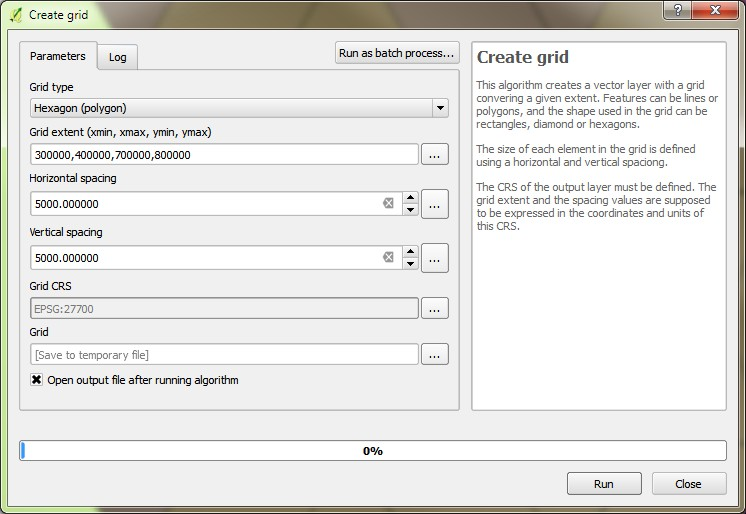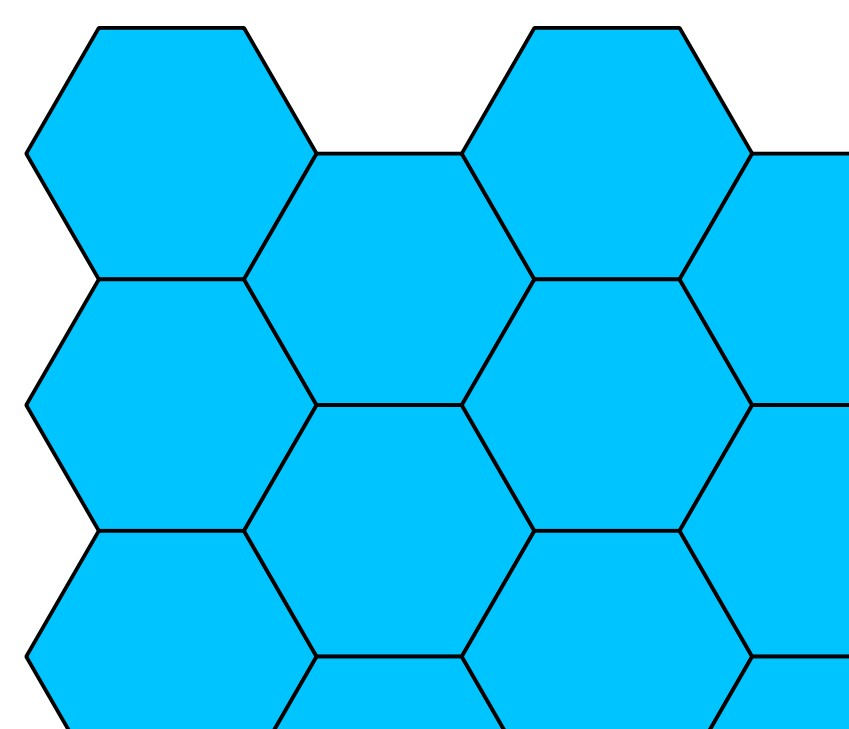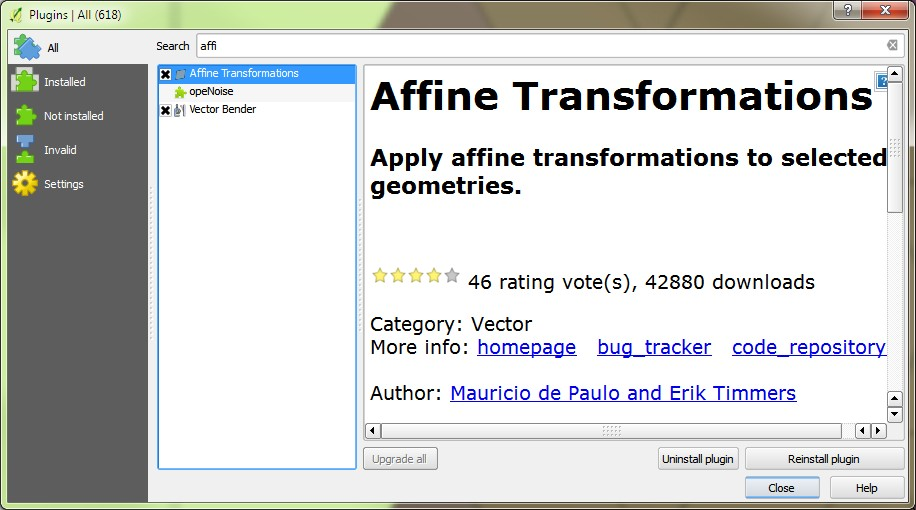# Hexperiments in QGIS

After seeing the post by John Nelson on hex binning using ArcGIS I thought I better try it in QGIS as the effect is pretty neat.

Open the Processing toolbox and find the QGIS geoalgorithm > Vector Creation Tools > Create GridI created grid using 300000,400000,700000,800000 and cell size 5000, 5000. Note the order of the coordinates for the extents! Output as hex_grid_1.shpSave this hex grid layer as a new layer - hex_grid_2.shp Now you'll have two layers exactly the same.

QGIS creates a hex grid with flat tops and bottoms and pointy sides. I'll rotate this later on to get the pointy bits top and bottom.

Add the QGIS Affine Transformation plugin to QGIS if not already installed.Select all features in the layer hex_grid_2. Start an edit session.

Open the Affine Transformation plugin, select the hex_grid_2 layer and choose to transform the whole layer.In the transformation matrix set the parameters like this:

``````X' = 1x + 0y + -1443.37567297
Y' = 0x + 1y + 2500.0
``````

How did I get these numbers? Well, leave the four cells on the left at their default values of 1 and 0. We only want to change the two cells on the right to shift the layer in the XY plane. My hex grid had a cell size of 5000, 5000 and so my shift in the X direction needs to be half the distance between two parallel sides: 5000/2 = 2500. My shift in the Y direction is 1/4 of the distance between points on a hex cell. This shift of -1443 and 2500 will move the cells and align the vertices of the cells perfectly to create a pseudo 3D effect.

The attribute table of the hex grid layer has values for top, bottom, left and right for each cell. So, if you have a different cell size to mine, just divide the difference between top and bottom by two and the difference between left and right by four to get your parameter values.

Your layer will shift. Check it is in the correct place, save edits and stop editing.Now we need to intersect these two layers to create a final layer of hex bins with internal partitions.Do your point in polygon count, style 'em up and see what you get!

#### Rotating to get the pointy bits up

Now, if you want to rotate your hex grid layer so the pointy bits are top and bottom you need to fiddle with the Affine Transformation plugin and work out how to rotate it 90 degrees around the layer centre point.

After some searching I found the solution on hydrogeotools.

This is what I used:

``````x' = cos(θ)*x -sin(θ)*y + (x0 - cos(θ) * x0 + sin(θ) * y0)
y' = -sin(θ)*x + cos(θ)*y + (y0 - sin(θ) * x0 - cos(θ) * y0)
``````

Caveat: Theta (θ) needs to be in radians and not degrees and that had me scratching my head for a bit. To rotate 90 degrees, convert to radians with `pi * 90 / 180` and you'll get `1.570796`. And `x0` and `y0` are the coordinates of the point you want to rotate around.

Thus, the parameters to be fed into the Affine plugin are:

``````x' = a, b, c = cos(θ), -sin(θ), (x0 - cos(θ) * x0 + sin(θ) * y0)
y' = d, e, f = -sin(θ), cos(θ), (y0 - sin(θ) * x0 - cos(θ) * y0)
``````

which in my case resulted in

``````x' = 0, -1, 1100000
y' = 1, 0, 400000
``````

Bung those in the transformer and the layer should rotate 90 degrees around 350000, 750000.

I also did it in PostGIS with the ST_Rotate function (a lot easier but then I did have a handy PostGIS DB nearby):

``````CREATE OR REPLACE VIEW hexgrid1_rotate AS
SELECT id, ST_Rotate(geometry, -pi()/2, 350000, 750000) FROM hexgrid1;
``````

I loaded my shapefile layer into the database and created a rotated view of it. The SQL above takes the geometry, rotates it 90 degrees (converting to radians) clockwise around a point (350000,750000). I added this into QGIS, saved is as a shapefile, saved it again and went through the affining process (same as above but remember your X and Y shifts are now swapped because you've rotated your layer) to shift the layer into the correct position.As ever, there may well be a better way of doing this (maybe I should learn some Python...) and thank you to the giants with big shoulders...